Next: GETTING THE WAVES FROM Up: Layers revealed by scattered Previous: REFLECTION AND TRANSMISSION COEFFICIENTS

# ENERGY FLUX IN LAYERED MEDIA

First consider wave resonance in a layer. Let the travel time through the layer and back again be given by the delay operator Z. The situation is shown in Figure 4. The wave seen above the layer has the form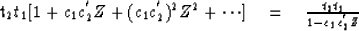It is no accident that the infinite series may be summed. We will soon see that for n layers the waves, which are of infinite duration, may be expressed as simple polynomials of degree n. We will consider many layers and the general problem of determining waves given reflection coefficients and determining reflection coefficients given waves.

 8-4 Figure 4 Some rays corresponding to resonance in a layer.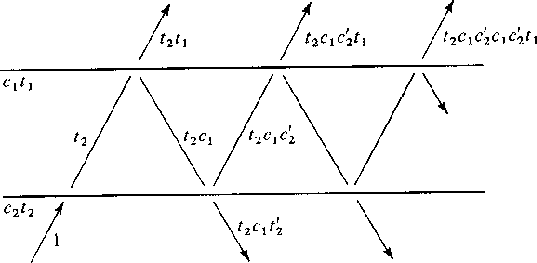The reflection and transmission coefficients show one how to calculate the waves resulting from a wave impinging on a layer. Equation (11) relates to Figure 5 and shows how from the waves U an D' one extrapolates into the future to get U' and D.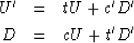(11)

 8-5 Figure 5 Waves incident and reflected from an interface.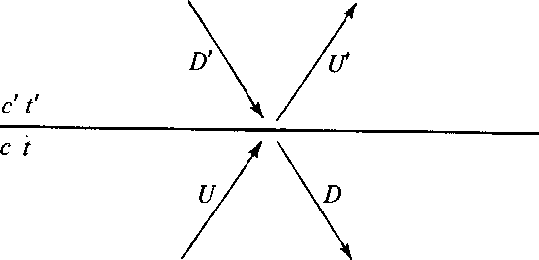Let us rearrange (11) to get U' and D' on the right and U and D on the left. Then we will have an equation which extrapolates from the primed medium to the unprimed medium. We get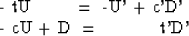which may be arranged in the matrix form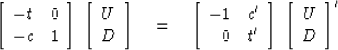Now premultiplying by the inverse of the left-hand matrix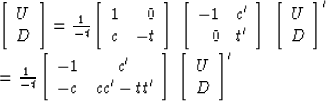finally getting the result, an equation to extrapolate from the primed medium to the unprimed medium.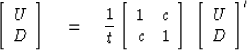(12)

Now let us consider the Goupillaud type layered medium shown in Figure 6. For this arrangement of layers, (12) may be written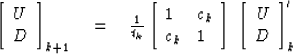8-6 Figure 6 Goupillaud-type layered medium (layers have equal travel time).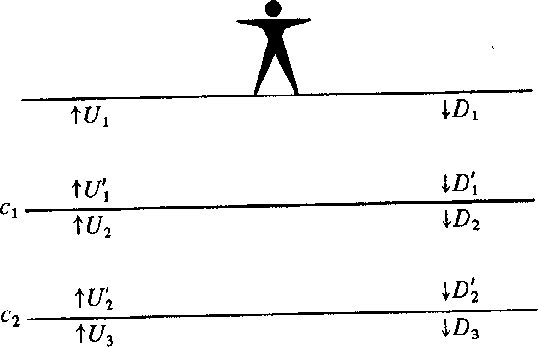Let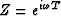where T, the two-way travel time, equals the data sampling interval. Clearly, multiplication by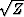is equivalent to delaying a function by T/2, the travel time across a layer. This gives in the kth layer a relation between primed and unprimed waves.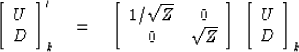(13)

Inserting (13) into (12) we get a layer matrix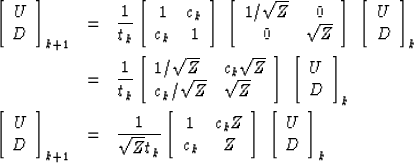(14)

If there is energy flowing through a stack of layers, there must be the same total flow through the kth layer as through the (k + 1)st layer. Otherwise, there is an energy sink or source at the layer boundary. The net upward flow of energy (energy flux) at any frequency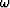in the kth layer is given by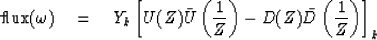(15)

To establish that this is indeed independent of k, we take the Hermitian conjugate (transpose and conjugate with respect to real)of (14).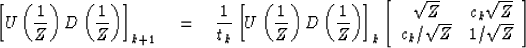(16)

Now combine (14) with (16) in the form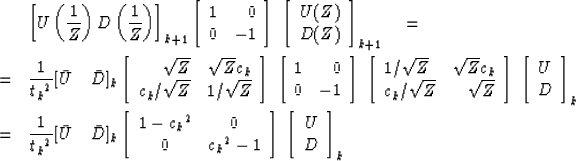(17)

Since (1 - ck2)/tk2 = t'k/tk = Yk /Yk+1 this may be rewritten as the desired result, namely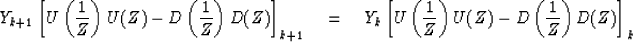(18)

Equation (18) says that at each frequencythe energy flowing through the kth layer equals the energy flowing through the (k + 1)st layer.

 8-7 Figure 7 Basic reflection seismology geometry. The person initiates an impulse going downward. The earth sends back -R(Z) to the surface. Since the surface is perfectly reflective, the surface sends R(Z) back into the earth. Escaping from the bottom of the layers is a wave E(Z) which is heading toward the other side of the earth.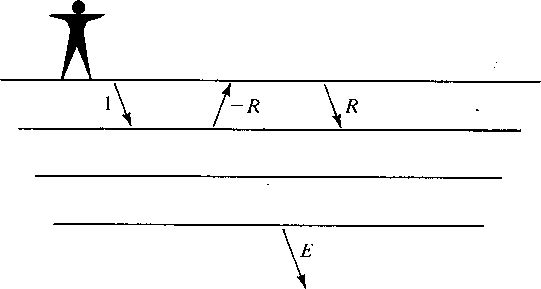This energy flux theorem leads quickly to some sweeping statements about the waveforms scattered from layered structures. Figure 7 shows the basic geometry of reflection seismology. Applying the energy flux theorem to this geometry we may say that the energy flux in the top layer equals that in the lower halfspace so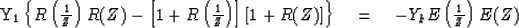or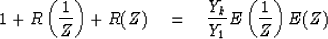(19)

This very remarkable result says that if we were to observe the escaping wave E(Z), we could by autocorrelation construct the waveform seen at the surface. We will later see that E(Z) is minimum-phase so that E could be constructed from R by spectral factorization.

Now let us turn our attention to the earthquake seismology geometry depicted in Figure 8. Applying the energy flux theorem to this geometry we obtain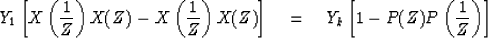or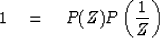(20)

The interpretation of the result is that the backscattered waveform P(Z) has the form of an all-pass filter. This result may have been anticipated on physical grounds since all the energy which is incident is ultimately reflected without attenuation; thus the only thing which can happen is that there will be frequency-dependent delay.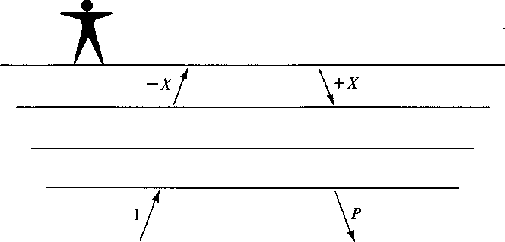8-8
Figure 8
Earthquake seismology geometry. An impulse 1 is incident from below. The waveform X(Z) is incident upon the free surface and is reflected back down. The waveform P(Z) scatters back into earth.Finally, we will derive a theorem which relates energy flux to impedance and admittance functions (these functions have Fourier transforms with a positive real part). Suppose that a downgoing wave D(Z) is stronger than an upgoing wave U(Z) at all frequencies, i.e.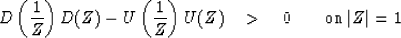(21)

(Note that this does not imply |dt| > |ut|.) We will abbreviate (21) by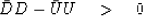(22)

From (21) or (22) we will deduce that (D-U)/(D+U) has a Fourier transform with a positive real part. We have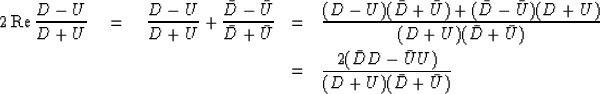(23)

The numerator of (23) is positive by hypothesis (22) and the denominator of (23) is positive, since it is the spectrum of the time function dt + ut and any spectrum is always positive. Thus (D - U)/(D + U) is called positive real." The acoustical interpretation of (D - U)/(D + U) is that (D - U) represents the vertical component of material velocity and (D + U) represents the material pressure.Next: GETTING THE WAVES FROM Up: Layers revealed by scattered Previous: REFLECTION AND TRANSMISSION COEFFICIENTS
Stanford Exploration Project
10/30/1997